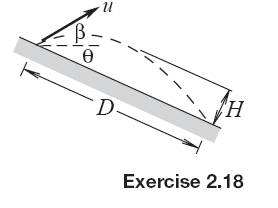### Create an Account

Home / Questions / A ball is thrown down an incline whose angle of elevation is θ The initial velocity is u a...

# A ball is thrown down an incline whose angle of elevation is θ The initial velocity is u at an angle of elevation β Derive an expression for the distance D measured along the incline at which the

A ball is thrown down an incline whose angle of elevation is θ. The initial velocity is u at an angle of elevation β. Derive an expression for the distance D measured along the incline at which the ball will return to the incline. Also determine the maximum height H, measured perpendicularly to the incline, of the trajectory, and the corresponding velocity of the ball at that position.Jun 18 2020 View more View LessSubscribe To Get Solution